# 4. Optimization for Technology CAD

Man darf nicht das, was uns unwahrscheinlich und unnatürlich

erscheint, mit dem verwechseln, was absolut unmöglich ist.''

Carl Friedrich Gauß4.1

THIS chapter first discusses the different optimization techniques and strategies that are commonly used in modern optimization applications. The second part of this chapter deals with the industrial requirements for optimization as well as its challenges for TCAD applications. The third part shows the need of an optimization framework and the resulting concepts which fulfills the presented requirements.

In this work the term optimization is used as a search for a minimal or maximal value for an objective function (also called score function) within certain defined constraints. It is a widely used practice that optimization problems are formulated as a minimization task of an objective score function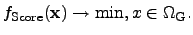(4.1)

To perform a maximum search, the formalism can be transformed to a minimum search for negative values of an objective score function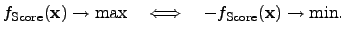(4.2)

Despite of the different score functions for the optimization, the mathematical convergence criteria for both optimization algorithms remain valid 4.2. The optimization problems discussed in this thesis are finite-dimensional optimizations of the following type: A given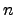-dimensional variable vector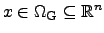of an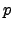-dimensional objective score function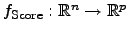has to be optimized globally in order to obtain a resulting vector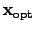which minimizes the value of the score function in a certain domain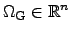. Equation (4.1) can also be expressed by using the following equivalent notation: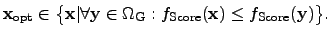(4.3)

In this definition, the function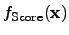denotes a continuous objective score function which has to be minimized. To determine a mathematically suitable criterion for minimization, the objective functionhas to apply a metric which maps the simulation result in a scalar-valued quantity.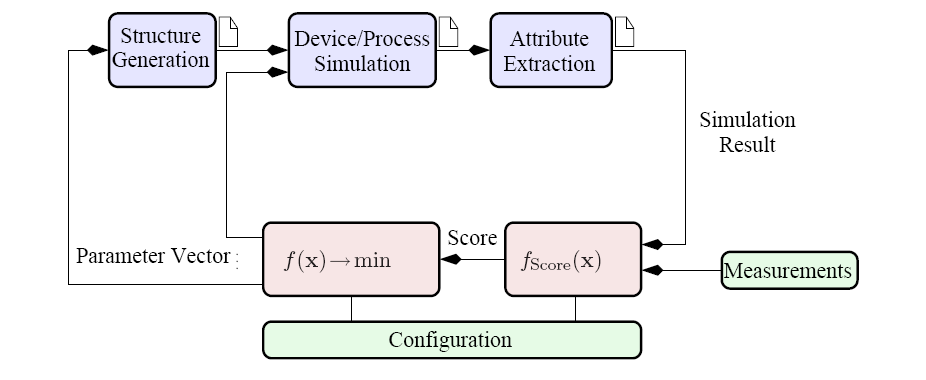The industrial requirements requires an objective score function of the form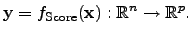(4.4)

However, this type of optimization would require optimization tools that are capable to operate with PARETO4.3 sets which is not commonly available in optimization tools. To overcome this kind of problem, a weighted norm can be applied to the score function and reads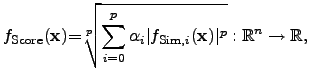(4.5)

where the result of this score functions is a real number, which can be optimized using the property that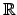is an ordered field. If the weight parameters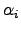are set to 1, (4.5) can be written as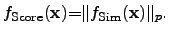(4.6)

In typical TCAD applications such objective score functions represent sequences of simulation software tools and thereforecan also include some necessary post-processing steps. A typical data flow of an optimization run is shown in Figure 4.1 where certain different input parameters can be applied to each simulation tool separately. In this depicted example, the simulation flow consists of a tool for the generation of the device geometry, the device simulator, and at the end a tool for the extraction of objective parameters from a finite-dimensional simulation result in order to compare it with reference data, which can be either constant values, analytical functions, or quantities in tables obtained from measurements.

In order to limit the optimum search to a certain domain and to weight or exclude certain parameter constellations, the input parameter space can be constrained by lower and upper bounds as well as by a finite number of constraint functions. In the following chapter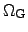denotes a convex4.4 and closed finite-dimensional domain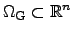which can be further constrained by functions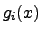which yields in the most general case a non-convex shape and can be expressed as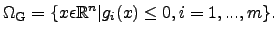(4.7)

The constraint functionscan map for instance some physical constraints to the input parameter domain, represent some technological or economical constraint from the fabrication processes, or these functions can be used to avoid parameter constellations which are not allowed, either by specifications or due to patent laws. However, these functions have to be individually chosen for a particular optimization problem. Constraints forcan be applied a-priori in contrast to constraints for output parameters. Therefore, the resulting domain for valid output parameters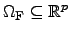is defined as the physical feasible values and the corresponding constraint function reads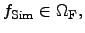(4.8)

where the valid values of the output domain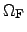are given by the nested function of the score functionapplied to the final results of the function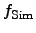which describes the sequence of the different simulation tools used.

While a-priori constraints limit the search domain only, a-posteriori constraints restrict the simulation results, which requires to calculate a complete simulation sequence to obtain a single result. This is thus very costly in time. Therefore, one tries to transform the constraints for the output in constraints for input parameters.

For some cases, where the constraints of simulation results have to be included into the constraint functions, an estimation can be performed to approximate the simulation results in advance. If the calculations of the original function is very time-consuming compared to the time for evaluating the approximation, this method provides the benefit of saving time by excluding certain a-priori known not valid simulation results.

With the domains (4.7) and (4.8) the initially constrained minimization problem (4.1) can be reformulated by using barrier or penalty functions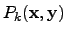in order to obtain an unconstrained surrogate optimization problem [219,220].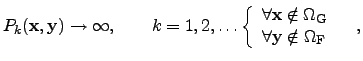(4.9)

which provide the possibility to use the original optimization framework with minor changes which can be specified by the user. In (4.9)symbolizes the input parameters andthe output parameters or the results of the simulation and the score function. The barrier and penalty functions try to account for the behavior of the outputas accurately as possible in order to save computation time. Then the penalty problem reads(4.10)

To conveniently apply such functions to a particular problem, the penalty function can be adapted to user defined constraints. For instance, there exist several different approaches for barrier and penalty terms. In the following, the barrier and penalty functions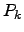are defined using a sequence of penalty parameters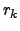, where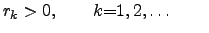and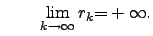(4.11)

Since the transition ofin (4.11) is numerically impossible, various finite approximations have been proposed in literature. However, the use of large numbers can result in serious convergence problems, because of the numerical calculations of the gradients when regions are considered which are located very close to the domain boundaries.

The formulation for barrier and penalty functions often considers a certain margin of the valid parameter domain. To prevent the search algorithm from moving too close to the domain boundary, a barrier function is applied that reaches the value infinity at the boundary. The penalty function charges a certain fine for the function if the search algorithm is outside of the specified domain. The inexact penalty functions have are vanishing inside the allowed domain. There are several methods to implement such barrier and penalty functions:

• The exact penalty function [221,222,223] vanishes inside the specified parameter domain and reaches a certain value greater zero outside the domain: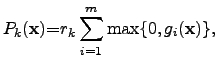(4.12)

where the corresponding constraint functions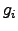apply with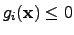inside the valid parameter domain and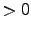outside.

• The exact quadratic penalty function [224,225] is quite similar to the previous one, but shows generally a quadratic increase with the distance from the domain boundary: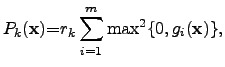(4.13)

where the corresponding constraint functionsapply. Again,inside the valid parameter domain andoutside.

• A logarithmic barrier function [226,227,228] offers the possibility of directing the parameter search to particular subdomains, in which the score function is superposed by a logarithmic function in the whole domain. Hence, the minimum of the sum of the score function and the logarithmic barrier function is located in the subdomain in which the optimum is. At the boundaries and outside the domain, the barrier function reaches infinity according to the definition of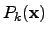: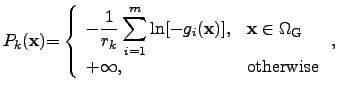(4.14)

where the corresponding constraint functionsapply forinside the valid parameter domain andoutside.

• With an inverse barrier function , the search region inside the parameter domain can be predefined similarly to the logarithmic barrier function, but with a differently shaped approximation: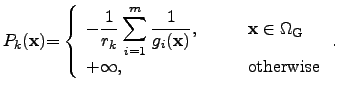(4.15)

Here, the constraint functionsare applied, whereinside the valid parameter domain andoutside.

• An inexact exponential penalty function  offers an efficient method to priorize a particular subdomain of the parameter domain without dealing with infinity. However, the value of the barrier function increases rapidly when the search algorithm leaves the valid parameter domain.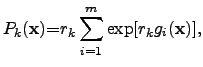(4.16)

where the constraint functions satisfyinside the valid parameter domain andoutside.
However, the user has always to choose the appropriate barrier or penalty functions in order to account for his particular needs and to check the convergence behavior of the whole optimization algorithm in advance. For instance if the score function and the contributions from the barrier and penalty function differ by many orders of magnitude, the discretization and gradient calculations algorithm of the optimizer might run into numerical problems in terms of precision and accuracy.

According to the principal behavior of the score function within the optimization problem, an appropriate barrier or penalty function modifies the original optimization problem in the same way as the score function would, but provides an additional term to the score function which allows to exclude certain domains from the original parameter space or to priorize certain subdomains for example if several optimal values are expected. Since many of the available optimization strategies do not inherently support constraint functions, additional barrier and penalty function are often used in non-linear optimization problems where only a certain set of optimization strategies are available for utilization with in a framework.

Subsections
Stefan Holzer 2007-11-19